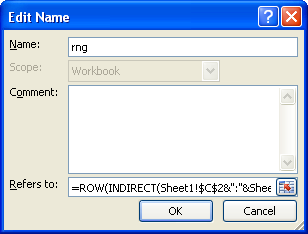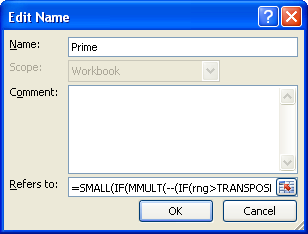# How to generate prime numbers with Excel

Scenario

Suppose you are asked to find out all the prime numbers in an interval using MS Excel.Suppose lower limit of a range is entered in cell C2 and upper limit in cell C3.
Steps to generate prime numbers with Excel
1. Press CTRL + F3 to open the Name Manager.
2. Click on "New".
3. In the "Name" field, type rng
4. In the "Refers to:" box, enter =ROW(INDIRECT(Sheet1!\$C\$2&":"&Sheet1!\$C\$3))5. Click OK
6. Click "New"
7. In the "Name" field, type Prime
8. In the "Refers to:" box, enter:
```=SMALL(IF(MMULT(--(IF(rng>TRANSPOSE(rng-Sheet1!\$C\$2+2),MOD(rng,(rng>TRANSPOSE(rng-Sheet1!\$C\$2+2))*TRANSPOSE(rng-Sheet1!\$C\$2+2)))=0),rng-Sheet1!\$C\$2+2)=0,rng),ROW(INDIRECT("1:"&Sheet1!\$C\$3)))
```9. Click OK
10. Click Close

Excel 2007 or later versions :
Select cells C5:C25, enter =IFERROR(Prime,"")

Excel 2003 or earlier versions :
Select cells C5:C25, enter =IF(ISERROR(Prime),"",Prime)

Hit CTRL + SHIFT + ENTER to confirm this formula as an array formula. If done correctly, Excel will automatically place curly braces {...} around the formula.

Related Post :
Count prime numbers with Excel
Check if a number is prime with Excel
Related Posts
Share1.